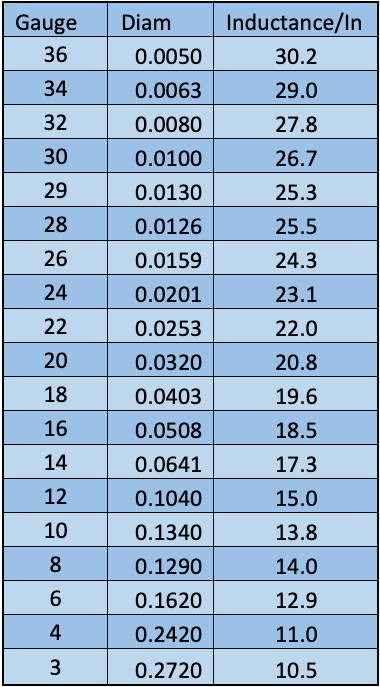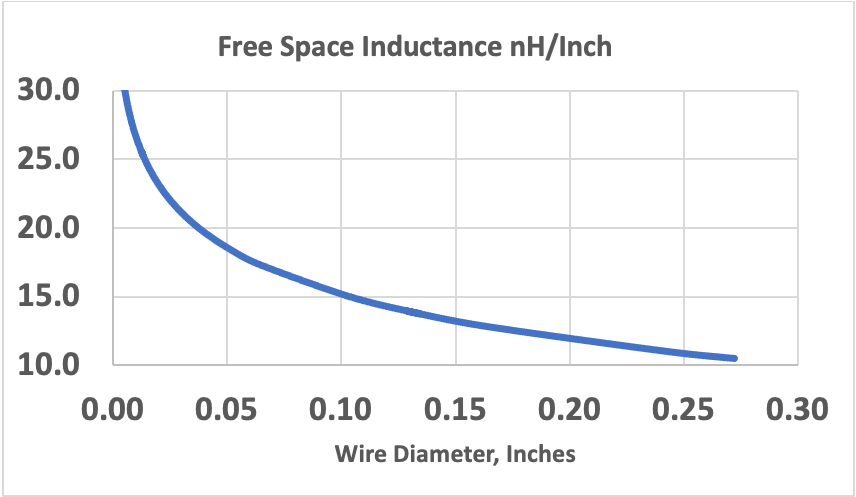|
|March 22, 2023

## What is the Inductance of a Straight Wire in Free Space?

Electronic circuits are often connected by wires. Small circuits may be connected by bond wires. A coaxial probe may have a short section of exposed center conductor, which is essentially a wire in free space. These all exhibit a certain amount of inductance. A chart of inductance of a straight wire in NanoHenrys per inch vs wire diameter or solid wire gauge is shown below in Table 1.Table 1 – Inductance of a Straight WireFigure 1 – Free Space Wire Diam vs Inductance/Inch

Choosing a value of 20 nH/In as a rule of thumb is reasonable.

For example, if a simple probe is made by exposing 0.2” of a semi-rigid cable center conductor and adding a ground return on the side of equal length, then the total inductance of the exposed conductor plus ground is about 8 nH. The reactance of this inductance reaches 50 at 6.25 GHz which would be the effective 3 dB bandwidth of the probe.

See this and more important radio frequency charts and formulas in this comprehensive application note